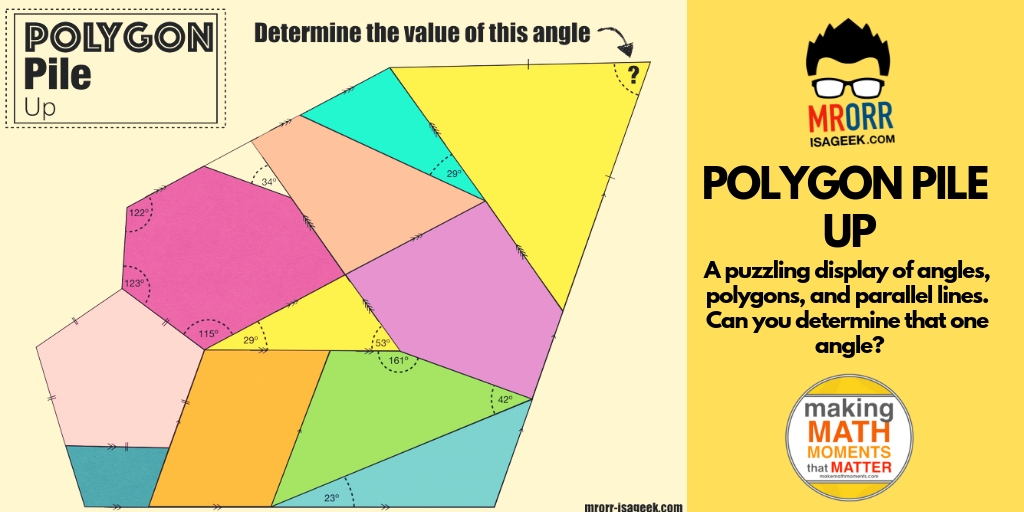# Polygon Pile Up

When it comes to angles involving parallel lines, triangles, and other polygons I’ve always assumed my grade 9 applied students “get this”. I’ve felt that angles were an easy topic. I guess I thought this because most students seem pretty happy when solving angle problems and for the most part being doing pretty well on assessments. However, this year I noticed two inadequacies that I am trying to address.

1. Most of my students didn’t actually know what an angle measurement of 65 degrees really means.
2. They have a hard time determining what information is needed when solving multi-step angle problems. Lack of a good strategy.

When having students determine angles in triangles almost all of them knew that all three angles should add to 180 degrees. The trouble came when I saw some answers like this (from more than one student).What bothered me was the location of the 40. I wondered why outside the triangle? I pressed this student for more info. I asked him to draw me any right triangle and label the three angles.Hmmm…I asked him to point to one of the angles. He pointed to where he labeled the 85. What I found is that this student was mixing up length measurements with rotational measurements and he was not alone.

I found a great activity to hit this head on. Laser Challenge from Desmos worked wonders to get my students to understand and experience rotational measurements. Students have to enter values to rotate the laser and mirror to hit targets.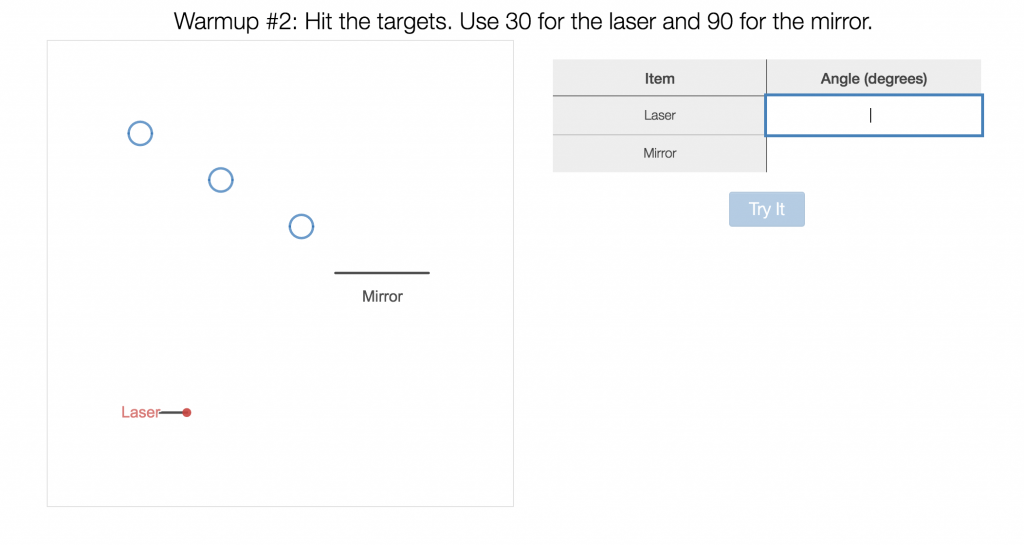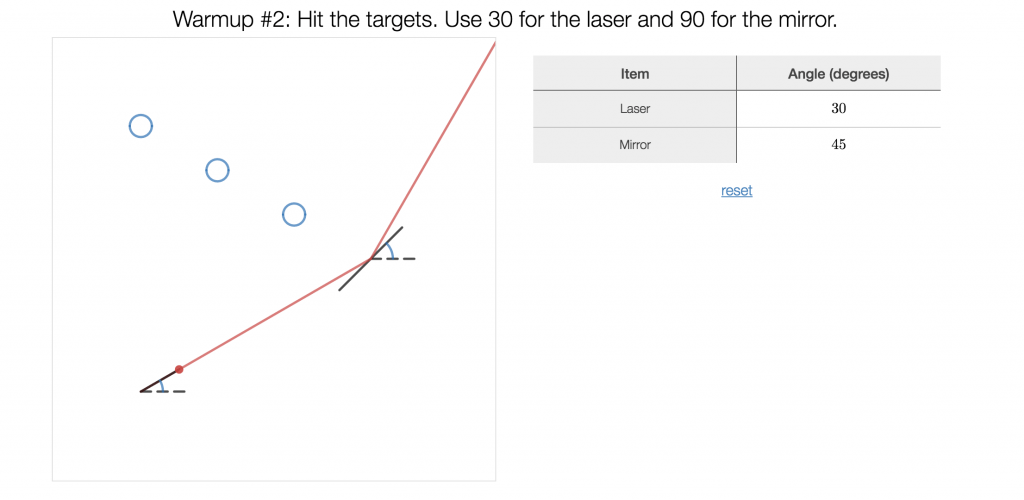My students “felt” what 60 degrees is. Experiencing that rotation made all the difference to clear up what we were actually measuring. When second semester rolled around and my new crop of kids came in we started with this activity right away.

Most of our students struggle with solving complex problems where they have to think of a strategy. Before I gave them something like this,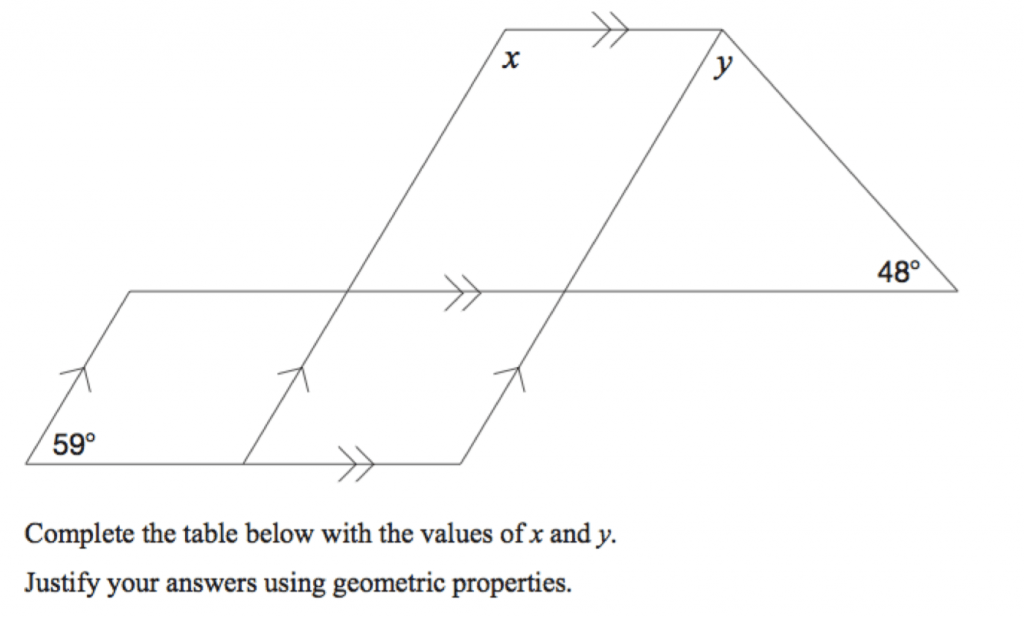I wanted to them to experience what information would be useful to know first. I decided to turn the problem around and inside out.

I gave them this.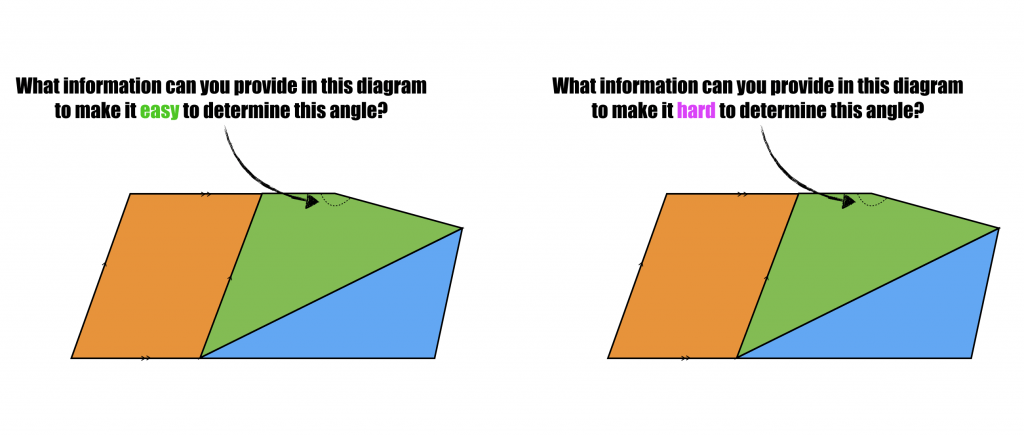I wanted them to think backwards….just like we need to do sometimes when solving longer problems. On the “easy” side most filled in 3 angles in the quadrilateral. What was great was that prepared them to think what we could leave out for the harder one. This simpler diagram challenged my class to think, plan, and strategize!

It was great to do this before we introduced this puzzle Jim Roesch, Kristyn Wilson, and myself created:

[There is a video embedded here — Can’t see it? Click through to the post page]

Here is the puzzle

And to really challenge yourself or your students here is a blank one. Can you fill it in so it’s “hard” to determine that indicated angle? What is the least amount of info you can give to bring out the most amount of thinking? Share them out!# From Circles to Polygons

My previous attempts at teaching the sum of interior angles in a polygon with grade 9 applied students went like this:

1. Day 1: Teach the sum of interior angles in a triangle. +practice problems
2. Day 2: Teach the sum of interior angles in a quadrilateral. +practice problems
3. Day 3: Use the pattern of triangles in a polygon to determine the formula for the sum of interior angles in a polygon. +practice problems.

I taught those lessons as if each is not related to the next!

Also, those lessons above had me doing most of the talking. I convinced myself that I was doing a discovery lesson with them…but I was really just showing/demonstrating to them that the angles added up to 180 or 360, etc

I wanted more doing!

Here was our progression this time around,

### Day 1: Circles to Angles

I showed this gif animation (not what I really wanted but I thought it would do the job).Then independently I had them answer: What do you notice? and, What do you wonder? After 2 minutes they shared with their partner. After 1 more minute they shared with the class.We used their noticing and wondering a to have a discussion on polygons, regular polygons, and acute vs. obtuse angles.

I posed the question:

“What would the interior angle be in a regular polygon if it had 20 sides, 30, 50?

I emphasized that looking for patterns can help us generalize. We set out on a path to find out what the sum of the interior angles for 3,4,5,6,7,8 sided figures…maybe we can see a pattern.
I handed out a page with 8 circles of varying sizes.I asked them to close their eyes and randomly plop their finger down on a circle.

I asked them to place a point randomly on the circumference of the circle. And then to add two more anywhere on the circle.

We connected the points up…at which time we talked about convex polygons.I handed out protractors. Measure the angles and write the sum inside the shape.

“How likely is it that any of drew the same triangle?”
Class: “None!”
I drew a giant triangle on the board and asked them to go up and put their sum inside the triangle.
Next they drew quadrilaterals, pentagons, hexagons, etc in the circles, measured angles, and calculated sums.By the end of day 1 our board looked like this.

###Day 2: Looking for Patterns

We looked at the sums placed in the shapes on the board for the triangle. There were lots close to 180 but not a lot exactly 180.

They seemed to remember that 180 was important and thought that all 3 angles should add to it.

I had them cut their triangle out. Cut each corner off…and try to arrange the pieces to make a half circle.

Boom! 180 degrees. On to the quadrilateral.

They guessed that the sum of the interior angles for a quadrilateral was 360 but weren’t sure why.
I asked them to pick a point on the quadrilateral and draw 1 line to make two triangles.

We agreed that each triangle’s interior angles add to 180 and there were 2 of them…then 2×180 is 360.
I handed out a sheet to keep track of the is potential pattern.We added those shapes to the sheet and plotted the points on the graph.

We divided the pentagon and remaining shapes into triangles and filled out the tableWe saw a definite pattern. A linear pattern.

As a class:

We found the first differences. We wrote a rule based on the number of triangles and related it to the number of sides in the polygon.

We then found the equation using the rate of change and y-intercept from the table.
We finally used the equation to determine the sum of interior angles for a 20 sided polygon…then found what the angles would be in a regular polygon.

To assess the students they completed practice problems on finding missing angles for varying shapes for the last 20 minutes while I circulated.

This course is all about patterns….use the patterns and prior knowledge to our advantage!